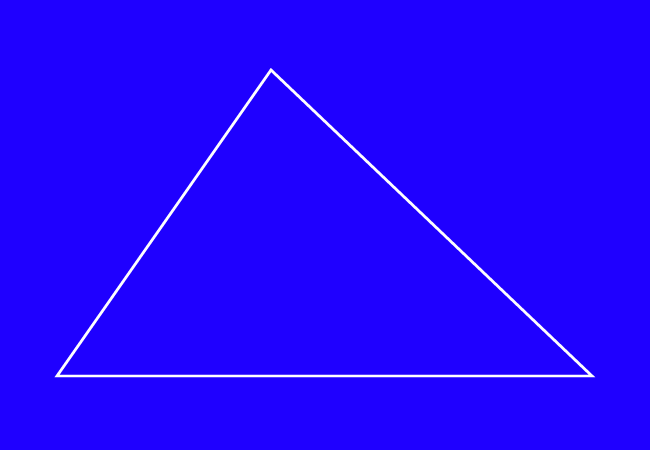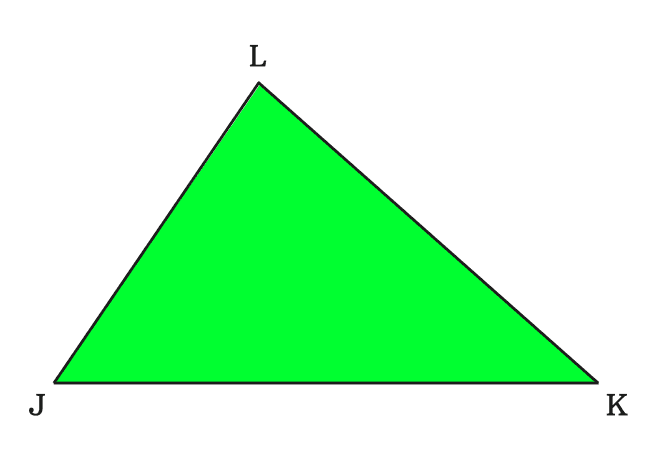# Sides of a Triangle

The line segments that form a triangle by connecting their endpoints as a closed geometric shape is called the sides of a triangle.

## IntroductionThe endpoints of three line segments can be connected as a closed geometric shape and it is called a triangle in geometry.

Each line segment acts as an edge or border of the triangle. In geometry, the edge or border is expressed by a word “side”. Hence, every line segment in any triangle is usually called as a side of the triangle.

There are three line segments in triangle. So, every triangle has three sides and their lengths can be equal or different.

### ExampleFirstly, let’s construct a triangle with three line segments. You can take equal or unequal sides for constructing it.

Now, create a closed geometric shape by joining an endpoint of one line segment to an endpoint of another line segment. It forms a triangle geometrically and it is called $\Delta JKL$ in this example.

In this triangle, the line segments act as a border or edge. Hence, the word “side” is used to express a line segment in geometry.

#### RepresentationMathematically, the line segments are written as $\overline{JK}$, $\overline{KL}$ and $\overline{LJ}$. In a triangle, the sides of triangle are line segments.

Therefore, the sides of the triangle are also written as $\overline{JK}$, $\overline{KL}$ and $\overline{LJ}$ mathematically.

Similarly, the lengths of sides of a triangle are also expressed as the way the lengths of line segments are expressed in mathematics.

Therefore, the lengths of the sides $\overline{JK}$, $\overline{KL}$ and $\overline{LJ}$ are written as $JK$, $KL$ and $LJ$ respectively.

Latest Math Topics
Jun 26, 2023
Jun 23, 2023

###### Math Questions

The math problems with solutions to learn how to solve a problem.

Learn solutions

Practice now

###### Math Videos

The math videos tutorials with visual graphics to learn every concept.

Watch now

###### Subscribe us

Get the latest math updates from the Math Doubts by subscribing us.